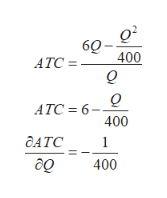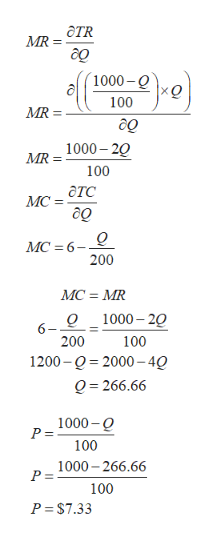# Consider a market with 190 consumers. Of these, 90 of them have individual (inverse) demands given by: PM(Q)=10−Q, while each of the other 100 has an individual (inverse) demand of PS(Q)=10−10Q. The cost function of the monopolist serving this market is C(Q) = 6Q - Q^2/400 . (a) Find the aggregate demand. Analyze the cost function and find what kind of returns to scale it exhibits. Compute the efficient total output (ignoring break-even constraints).(b) Compute the optimal linear price (and quantity) for this monopolist, and the deadweight loss.

Question
32 views
1. Consider a market with 190 consumers. Of these, 90 of them have individual (inverse) demands given by: PM(Q)=10−Q, while each of the other 100 has an individual (inverse) demand of PS(Q)=10−10Q. The cost function of the monopolist serving this market is
C(Q) = 6Q - Q^2/400 .
(a) Find the aggregate demand. Analyze the cost function and find what kind of returns to scale it exhibits. Compute the efficient total output (ignoring break-even constraints).
(b) Compute the optimal linear price (and quantity) for this monopolist, and the deadweight loss.
check_circle

Step 1

(a) Aggregate demand refers to the sum total of demand of all individual. It is provided that an individual inverse demand curve of 90 individual is PM(Q) = 10-Q and individual inverse demand curve of 100 individual is PS(Q)=10-10Q. Thus aggregate demand curve  is Q=1000-100P

Step 2

Returns to scale refers to the change in average total cost with an increase in output. total cost function of the monopolist firm is C(Q)=6Q-Q^2/400. By dividing the total cost function with Q, average total cost function can be achieved. since the first derivative of ATC is negative, this can be concluded that monopolist firm experiences increasing returns to scale.help_outlineImage Transcriptionclose60- 400 АТC - о о АТС 3 6- 400 ДАТС 1 до 400 fullscreen
Step 3

(b)  Optimal linear price and quantity can be obtained at a point where marginal revenue is exactly equal to marginal cost where MR is  ...help_outlineImage TranscriptioncloseaTR MR = до 1000-Q xо 100 MR MR = 1000-20 100 атс MС- до Q MC 6 200 MC = MR Q1000-20 6- 200 100 1200 0 2000-4Q Q 266.66 1000-0 P = 100 1000-266.66 P = 100 P \$7.33 fullscreen

### Want to see the full answer?

See Solution

#### Want to see this answer and more?

Solutions are written by subject experts who are available 24/7. Questions are typically answered within 1 hour.*

See Solution
*Response times may vary by subject and question.
Tagged in